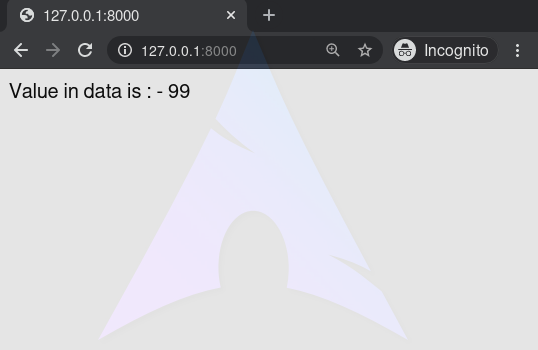GeeksforGeeks App
Open AppBrowser
Continue

# Boolean Operators – Django Template Tags

A Django template is a text document or a Python string marked-up using the Django template language. Django being a powerful Batteries included framework provides convenience to rendering data in a template. Django templates not only allow passing data from view to template, but also provides some limited features of a programming such as variables, for loops, comments, extends, if else etc.
This article revolves about how to use boolean operators in Templates. The {% if %} tag evaluates a variable, and if that variable is “true” (i.e. exists, is not empty, and is not a false boolean value) the contents of the block are output. One can use various boolean operators with Django If Template tag.

Syntax:

```{% if variable boolean_operator value %}
// statements
{% endif %}```

Example:
if tags may use and, or not to test a number of variables or to negate a given variable:

## html

 `{% if athlete_list and coach_list %}``    ``Both athletes and coaches are available.``{% endif %}` `{% if not athlete_list %}``    ``There are no athletes.``{% endif %}` `{% if athlete_list or coach_list %}``    ``There are some athletes or some coaches.``{% endif %}` `{% if not athlete_list or coach_list %}``    ``There are no athletes or there are some coaches.``{% endif %}` `{% if athlete_list and not coach_list %}``    ``There are some athletes and absolutely no coaches.``{% endif %}`

As one can see, the if tag may take one or several {% elif %} clauses, as well as an {% else %} clause that will be displayed if all previous conditions fail. These clauses are optional.

## Boolean operators – Django template Tags Explanation

Illustration of How to use Boolean operators in Django templates using an Example. Consider a project named geeksforgeeks having an app named geeks.

Refer to the following articles to check how to create a project and an app in Django.

Now create a view through which we will pass the context dictionary,
In geeks/views.py,

## Python3

 `# import Http Response from django``from` `django.shortcuts ``import` `render`` ` `# create a function``def` `geeks_view(request):``    ``# create a dictionary``    ``context ``=` `{``        ``"data"` `: ``99``,``    ``}``    ``# return response``    ``return` `render(request, ``"geeks.html"``, context)`

Create a url path to map to this view. In geeks/urls.py,

## Python3

 `from` `django.urls ``import` `path` `# importing views from views.py``from` `.views ``import` `geeks_view` `urlpatterns ``=` `[``    ``path('', geeks_view),``]`

Create a template in templates/geeks.html,

## html

 `{% if data == 99 %}``Value in data is : - {{ data }}``{% else %}``Data is empty``{% endif%}`

Let’s check what is displayed on “/” are displayed in the template.## Boolean Operators

== operator
Equality. Example:

```{% if somevar == "x" %}
This appears if variable somevar equals the string "x"
{% endif %}```

!= operator
Inequality. Example:

```{% if somevar != "x" %}
This appears if variable somevar does not equal the string "x",
{% endif %}```

< operator
Less than. Example:

```{% if somevar < 100 %}
This appears if variable somevar is less than 100.
{% endif %}```

> operator
Greater than. Example:

```{% if somevar > 0 %}
This appears if variable somevar is greater than 0.
{% endif %}```

<= operator
Less than or equal to. Example:

```{% if somevar <= 100 %}
This appears if variable somevar is less than 100 or equal to 100.
{% endif %}```

>= operator
Greater than or equal to. Example:

```{% if somevar >= 1 %}
This appears if variable somevar is greater than 1 or equal to 1.
{% endif %}```

in operator
Contained within. This operator is supported by many Python containers to test whether the given value is in the container. The following are some examples of how x in y will be interpreted:

```{% if "bc" in "abcdef" %}
This appears since "bc" is a substring of "abcdef"
{% endif %}```
```{% if "hello" in greetings %}
If greetings is a list or set, one element of which is the string
"hello", this will appear.
{% endif %}```
```{% if user in users %}
If users is a QuerySet, this will appear if user is an
instance that belongs to the QuerySet.
{% endif %}```

not in operator
Not contained within. This is the negation of the in operator.
is operator
Object identity. Tests if two values are the same object. Example:

```{% if somevar is True %}
This appears if and only if somevar is True.
{% endif %}

{% if somevar is None %}
This appears if somevar is None, or if somevar is not found in the context.
{% endif %}```

is not operator
Negated object identity. Tests if two values are not the same object. This is the negation of the is operator. Example:

```{% if somevar is not True %}
This appears if somevar is not True, or if somevar is not found in the
context.
{% endif %}

{% if somevar is not None %}
This appears if and only if somevar is not None.
{% endif %}```

My Personal Notes arrow_drop_up